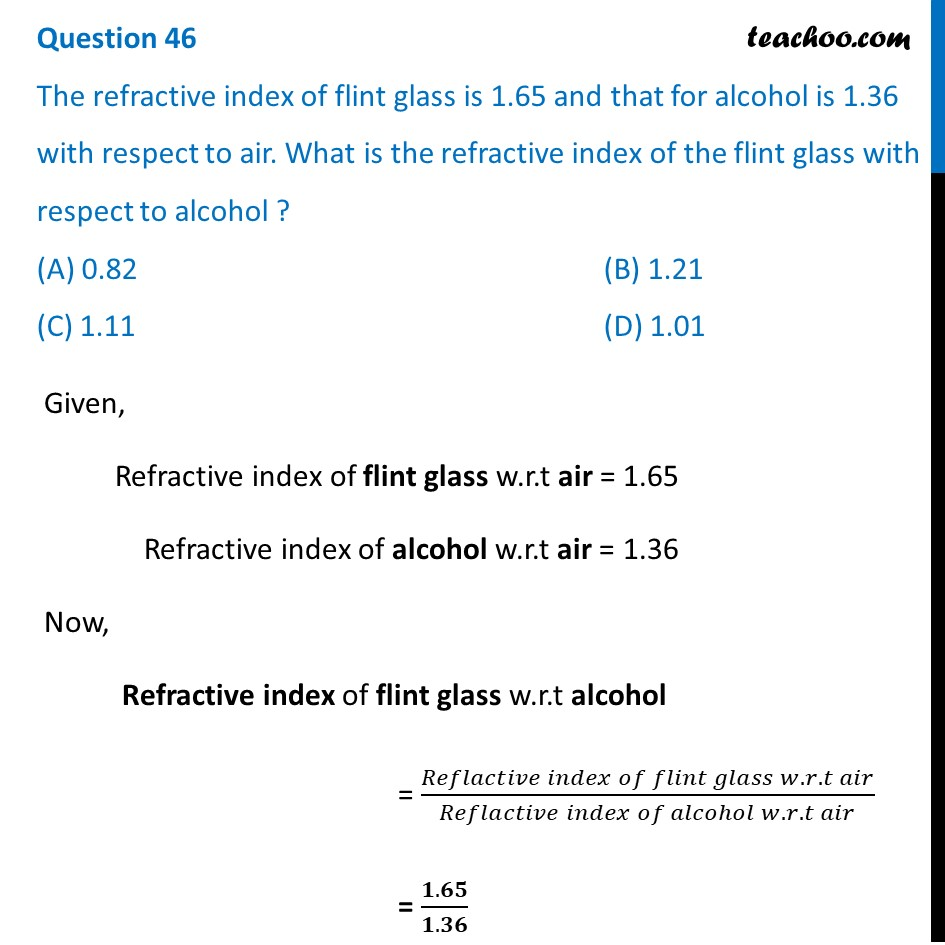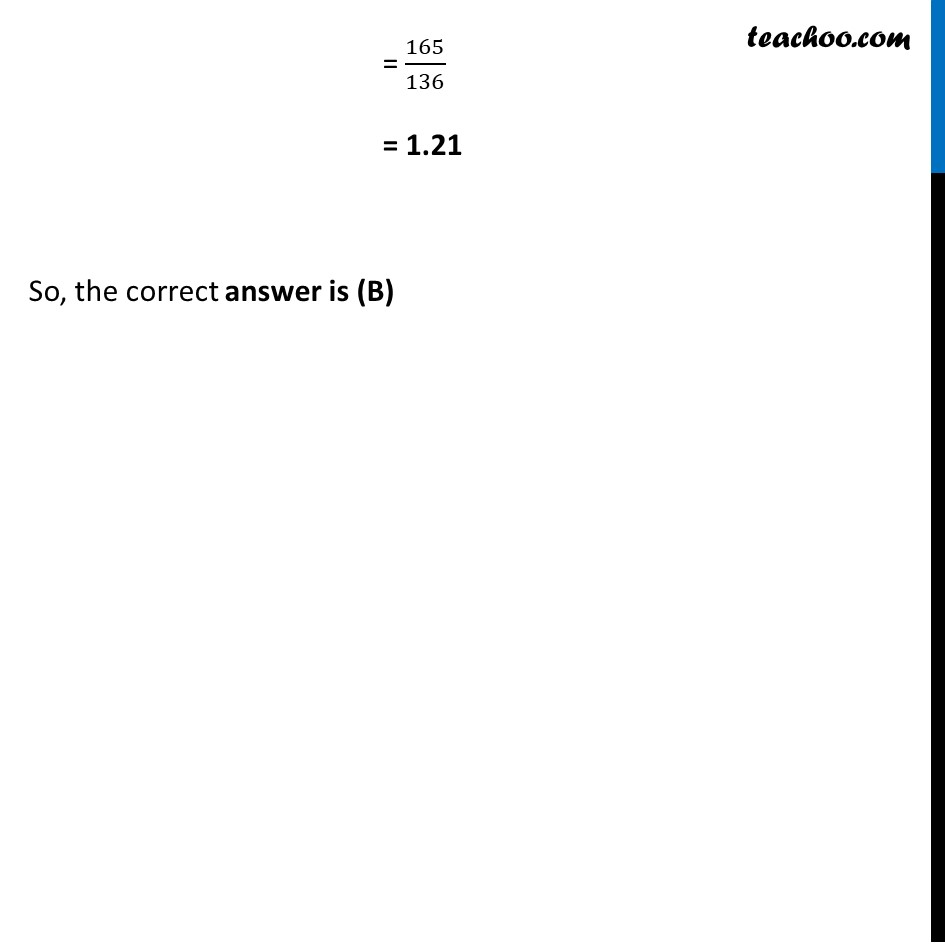Solutions - CBSE Class 10 Sample Paper for 2022 Boards - Science [MCQ]

Class 10
Solutions to CBSE Sample Paper - Science Class 10

## (D) 1.01Get live Maths 1-on-1 Classs - Class 6 to 12

### Transcript

Question 46 The refractive index of flint glass is 1.65 and that for alcohol is 1.36 with respect to air. What is the refractive index of the flint glass with respect to alcohol ? (A) 0.82 (B) 1.21 (C) 1.11 (D) 1.01 Given, Refractive index of flint glass w.r.t air = 1.65 Refractive index of alcohol w.r.t air = 1.36 Now, Refractive index of flint glass w.r.t alcohol = (𝑅𝑒𝑓𝑙𝑎𝑐𝑡𝑖𝑣𝑒 𝑖𝑛𝑑𝑒𝑥 𝑜𝑓 𝑓𝑙𝑖𝑛𝑡 𝑔𝑙𝑎𝑠𝑠 𝑤.𝑟.𝑡 𝑎𝑖𝑟)/(𝑅𝑒𝑓𝑙𝑎𝑐𝑡𝑖𝑣𝑒 𝑖𝑛𝑑𝑒𝑥 𝑜𝑓 𝑎𝑙𝑐𝑜ℎ𝑜𝑙 𝑤.𝑟.𝑡 𝑎𝑖𝑟) = (𝟏.𝟔𝟓)/(𝟏.𝟑𝟔) = 165/136 = 1.21 So, the correct answer is (B)